Question

# A metabolic reaction A to B is endergonic, with a standard free energy change of +0.5...

A metabolic reaction A to B is endergonic, with a standard free energy change of +0.5 kcal/mol. Describe two specific ways in which this reaction could be made favorable.

Two types of reactions : a) Exergonic reactions : An exergonic reaction is a reaction where the change in the free energy is negative indicating a spontaneous reaction.

b) Endergonic reaction : An  is a reaction in which the standard change in free energy is positive, and energy is absorbed. These kind of reactions are non spontaneous or unfavorable.

Two ways in which Endergonic reactions can be made favourable

1 )Endergonic reaction can occur by coupling them to another reaction which is exergonic more than them as in this case more than 0.5 kcal/ mol

For example biological reactions proceed via this method. Let assume this reaction below is

X. + Y ----------->. XY

too endergonic to occur. However it is made possible in the body by coupling it to a strongly exergonic reaction – such as the conversion of ATP into ADP and inorganic phosphate ions, ATP → ADP + Pi, so that

X. +. ATP. ----------->. ADP. +. XP​​​​​i

XP. +. Y. -------------->. XY. + P​​​​i

2)  If the following step of a Endergonic reaction is rapid and extremely Exergonic than that reaction can be pulled upto transition state because after that Exergonic reaction will take place.

#### Earn Coins

Coins can be redeemed for fabulous gifts.

Similar Homework Help Questions
• ### A reaction has a standard free-energy change of -11.40 kJ mol-1

A reaction has a standard free-energy change of -11.40 kJ mol-1(-2.725 kcal mol-1). Calculate the equilibrium constant for the reaction at 25°C.

• ### A reaction has a standard free-energy change of –16.50 kJ mol-1

A reaction has a standard free-energy change of –16.50 kJ mol-1(-3.944 kcal mol-1). Calculate the equilibrium constant for the reaction at 25 °C. Keq = _______

• ### The change in Gibbs free energy for an endergonic reaction is positive and is equal AG,...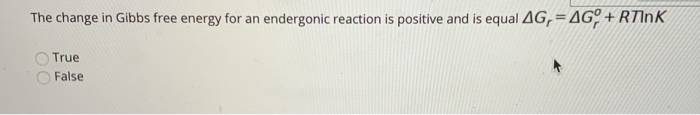The change in Gibbs free energy for an endergonic reaction is positive and is equal AG, = AG, + Rink True False

• ### Free Energy (kcal/mol) Reaction progress- Use the reaction energy diagram above to answer the following questions....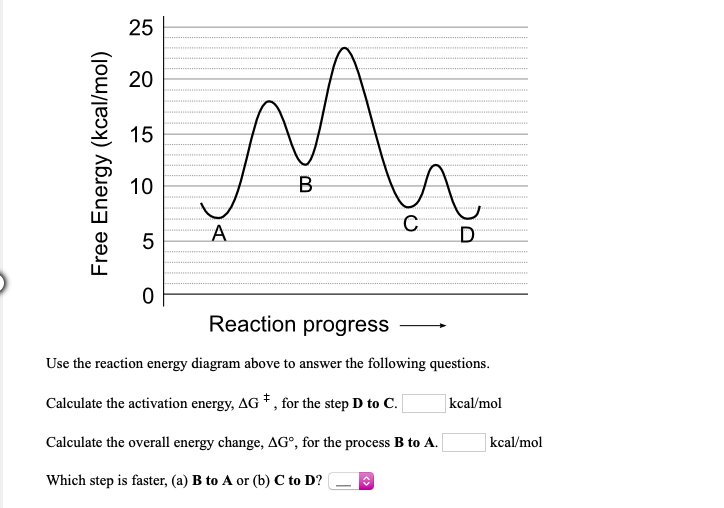Free Energy (kcal/mol) Reaction progress- Use the reaction energy diagram above to answer the following questions. Calculate the activation energy, AG * , for the step D to C. kcal/mol Calculate the overall energy change, AGⓇ, for the process B to A. kcal/mol Which step is faster, (a) B to A or (b) C to D? - Free Energy (kcal/mol) Reaction progress - Use the reaction energy diagram above to answer the following questions. Calculate the activation energy, AG ,...

• ### Calculate the change in Gibbs Free Energy (AG) for the following system. Is this reaction endergonic...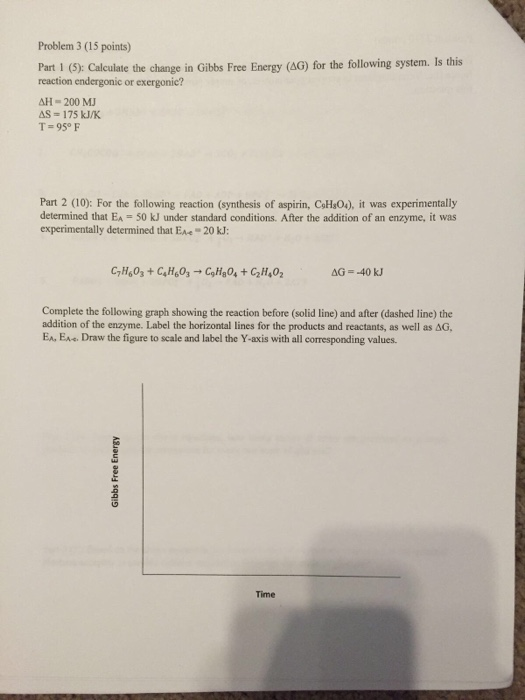Calculate the change in Gibbs Free Energy (AG) for the following system. Is this reaction endergonic or exergonic? For the following reaction (synthesis of aspirin. C_5H_3O_4), it was experimentally determined that Ea = 50 kJ under standard conditions. After the addition of an enzyme, it was experimentally determined that E_aa = 20 kJ: Complete the following graph showing the reaction before (solid line) and after (dashed line) the addition of the enzyme. Label the horizontal lines for the products and...

• ### presente Organic Chemistry oudon The standard free energy of activation of one reaction A is 93.80...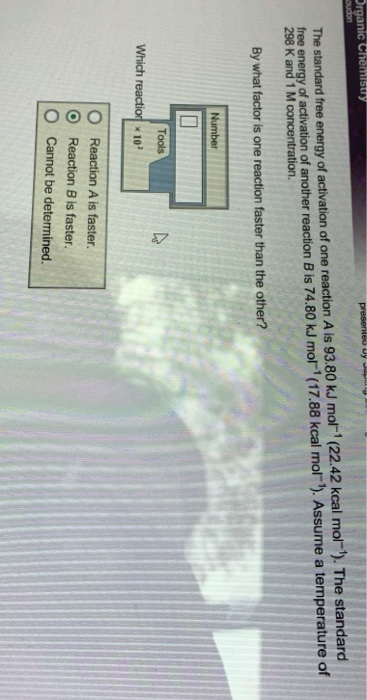presente Organic Chemistry oudon The standard free energy of activation of one reaction A is 93.80 kJ mol-'(22.42 kcal mol-'). The standard free energy of activation of another reaction B is 74.80 kJ mol-'(17.88 kcal mol). Assume a temperature of 298 K and 1 M concentration. By what factor is one reaction faster than the other? Number Tools 10 Which reactior O Reaction A is faster. Reaction B is faster. Cannot be determined. O

• ### 2. Define the following terms: a. exergonic b. endergonic c. entropy d. enthalpy e. free energy f. metabolic flux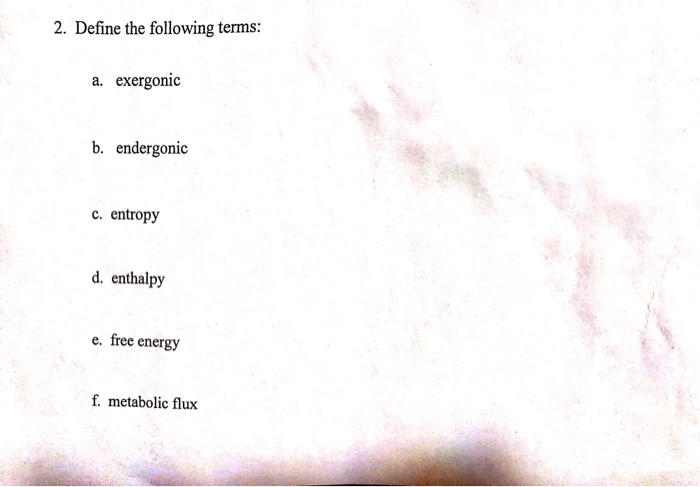2. Define the following terms: a. exergonic b. endergonic c. entropy d. enthalpy e. free energy f. metabolic flux

• ### The standard free energy of activation of a reaction A is 71.6 kJ mol–1 (17.1 kcal...

The standard free energy of activation of a reaction A is 71.6 kJ mol–1 (17.1 kcal mol–1) at 298 K. Reaction B is one million times faster than reaction A at the same temperature. The products of each reaction are 10.0 kJ mol–1 (2.39 kcal mol–1) more stable than the reactants. (a) What is the standard free energy of activation of reaction B?

• ### The standard free energy of activation of a reaction A is 77.3 kJ mol–1 (18.5 kcal...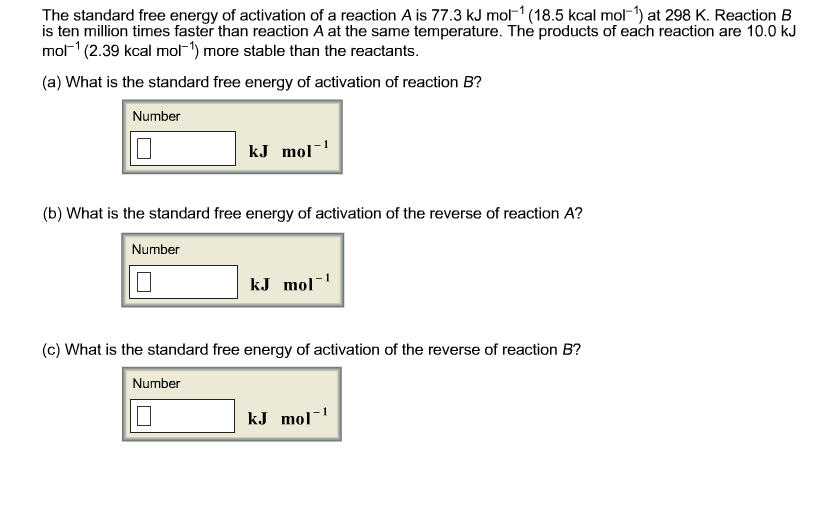The standard free energy of activation of a reaction A is 77.3 kJ mol–1 (18.5 kcal mol–1) at 298 K. Reaction B is ten million times faster than reaction A at the same temperature. The products of each reaction are 10.0 kJ mol–1 (2.39 kcal mol–1) more stable than the reactants. (a) What is the standard free energy of activation of reaction B?

• ### Free Energy (kcal/mol) Reaction progress Use the reaction energy diagram above to answer the following questions. C...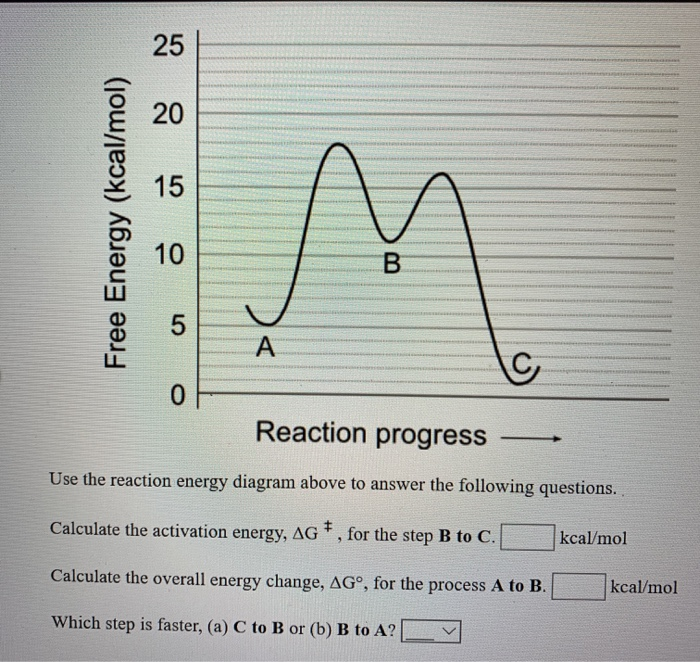Free Energy (kcal/mol) Reaction progress Use the reaction energy diagram above to answer the following questions. Calculate the activation energy, AG *, for the step B to C. kcal/mol Calculate the overall energy change, AG°, for the process A to B. kcal/mol Which step is faster, (a) C to B or (b) B to A?